Courses

# JEE(MAIN) Mathematics Mock Test - 2

## 30 Questions MCQ Test JEE Main Mock Test Series 2020 & Previous Year Papers | JEE(MAIN) Mathematics Mock Test - 2

Description
This mock test of JEE(MAIN) Mathematics Mock Test - 2 for JEE helps you for every JEE entrance exam. This contains 30 Multiple Choice Questions for JEE JEE(MAIN) Mathematics Mock Test - 2 (mcq) to study with solutions a complete question bank. The solved questions answers in this JEE(MAIN) Mathematics Mock Test - 2 quiz give you a good mix of easy questions and tough questions. JEE students definitely take this JEE(MAIN) Mathematics Mock Test - 2 exercise for a better result in the exam. You can find other JEE(MAIN) Mathematics Mock Test - 2 extra questions, long questions & short questions for JEE on EduRev as well by searching above.
QUESTION: 1

Solution:
QUESTION: 2

### If the equation [(k(x+1)2/3)]+[(y+2)2/4]=1 represents a circle, then k=

Solution: The given equation can be write as

=> 4k(x+1) ²+3(y+2) ²=12

on expanding wee get x² coefficient as 4k
and y² coefficient as 3
but in equation of circle x² coefficient is equal to y² coefficient

therefore 4k=3
=> k=3/4
QUESTION: 3

### The eccentric angles of the extremities of the latus-rectum intersecting positive x-axis of the ellipse ((x2/a2) + (y2/b2) = 1) are given by

Solution:
QUESTION: 4

If arg (z) = θ, then arg(z̅) =

Solution:
QUESTION: 5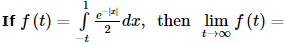Solution:
QUESTION: 6

If N N+ denotes the set of all positive integers and if f : NN+ → N is defined by f(n)    = the sum of positive divisors of (n)  then f (2k . 3), where k is a positive integer is

Solution:

f(2k. 3) = The sum of positive divisors of 2k . 3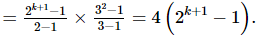QUESTION: 7

If a, b, c are different and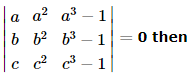Solution:

Explanation : A = {(a, a2, a3-1) (b, b2, b3-1) (c, c2, c3-1)}

=> {(a, a2, a3) (b, b2, b3) (c, c2, c3)} - {(a, a2, 1) (b, b2, 1) (c, c2, 1)} = 0

=> abc{(1, a, a2) (1, b, b2) (1, c, c2)} - {(a, a2, 1) (b, b2, 1) (c, c2, 1)} = 0

=> abc{(a, a2, 1) (b, b2, 1) (c, c2, 1)} - {(a, a2, 1) (b, b2 1) (c, c2, 1)} = 0

=> (abc-1){(a, a2, 1) (b, b2, 1) (c, c2, 1)} = 0

abc - 1 = 0

=> abc = 1

QUESTION: 8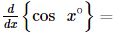Solution: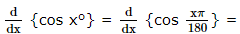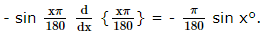QUESTION: 9

In the following question, a Statement of Assertion (A) is given followed by a corresponding Reason (R) just below it. Read the Statements carefully and mark the correct answer-
Assertion (A): Angle between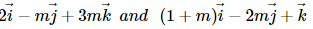is acute angle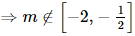Reason (R): If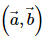is acute then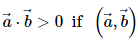is obtuse then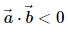Solution:
QUESTION: 10

The point on the curve y = x2 which is nearest to (3, 0) is

Solution:
QUESTION: 11

The degree of the differential equation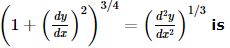Solution: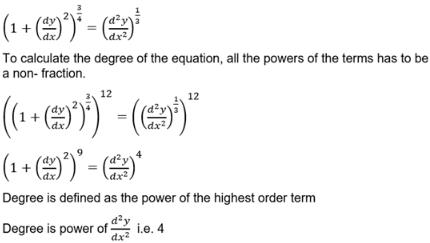QUESTION: 12

In the following question, a Statement of Assertion (A) is given followed by a corresponding Reason (R) just below it. Read the Statements carefully and mark the correct answer-
Assertion (A):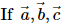are non zero vectors then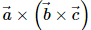is a vector perpendicular to all the vectors a → , b → , c →
Reason (R):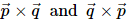are perpendicular to both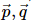Solution:
QUESTION: 13

If i2 = -1, then the sum i + i2 + i3 + ..... upto 1000 terms is equal to

Solution: There will equal n opposite signed terms that is 500 +ve one and 500-ve one therefore it's value comes to zero.
QUESTION: 14

A parallelogram is cut by two sets of m lines parallel to the sides, the number of parallelogram thus formed is

Solution:
Parallelogram is cut by two sets of m parallel lines to its sides.
then we have  2 sets of (m+2) parallel lines ( 2 lines of the parallelogram)
so parallelogram is formed by taking 2 lines from each set
= m+2C2 * m+2C2
= [(m+2)(m+1)/2 ]2
this also include 1 original parallelogram
so total number of new parallelogram formed is  =  (m + 2)2(m + 1)2/4
QUESTION: 15

If A and B are two events such that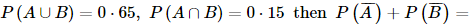Solution:
QUESTION: 16

The probability that a leap year will have exactly 52 Tuesdays is

Solution:

The probability of a year being a leap year is 1/4 and being non-leap is 3/4.A leap year has 366 days or 52 weeks and 2 odd days. The two odd days can be {Sunday,Monday},{Monday,Tuesday},{Tuesday,Wednesday}, Wednesday,Thursday},{Thursday,Friday},{Friday,Saturday},{Saturday,Sunday}.So there are 7 possibiliyies out of which 2 have a Sunday. So the probability of 53 Sundays in a leap year is 2/7.

So, the probability of 52 sundays is 1-2/7 = 5/7.

QUESTION: 17

Product of the real roots of the equation t2x2 + ∣x∣ + 9 = 0

Solution:
QUESTION: 18

If A.M. between two numbers is 5 and their G.M. is 4, then their H.M. is

Solution:

If x, y and z respectively represent AM, GM and HM between two numbers a and b, then
y2 = xz
Here x = 5, y = 4
then 16 = 5 x z
z = 16/5

QUESTION: 19

If the coefficient of correlation between x and y is 0.28, covariance between x and y is 7.6, and the variance of x is 9, then the standard deviation of the y series is

Solution: N the given problem it is SD of x is 3: (or Variance of x is 9). As Variance = (Sx)^2. We know the relation : correlation coefficient (r) = Cov (x,y) / (Sx * Sy) so, 0.28 = 7.6 / (3 * Sy) From here we get the value of SD of Y : Sy = 9.05.
QUESTION: 20

The equation line passing through the point P(1,2) whose portion cut by axes is bisected at P, is

Solution: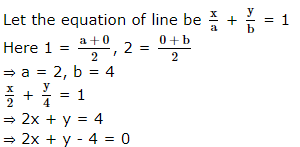QUESTION: 21

The equation of the plane through intersection of planes x + 2y + 3z = 4 and 2x + y - z = -5 and perpendicular to the plane 5x + 3y + 6z + 8 = 0 is

Solution:
QUESTION: 22

The projections of a line segment on the co-ordinate axes are 12,4,3 respectively. The length of the line segment is

Solution: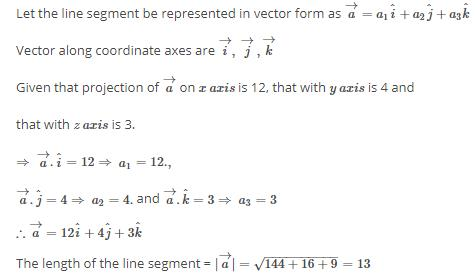QUESTION: 23

sin 120° cos 150° − cos 240° sin 330° =

Solution:

Sin120= sin(90+30)=cos30= sqrt(3)/2

Cos150= cos(180–30)= - cos30= -sqrt(3)/2

Cos240= cos(180+60)= - cos60= -1/2

Sin330= sin(360–30)= - sin30= - 1/2

((Sqrt(3)/2) * (-sqrt(3)/2))-((-1/2) * (- 1/2))

= (- 3/4)-(1/4)

{ since ‘- ‘multiplied with ‘+’ equals ‘-’ ,

And ‘-’ multiplied with ‘-’ gives ‘+’}

=-1

Therefore the answer is - 1

QUESTION: 24

Let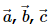be three non-coplanar vectors such and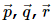be vectors defined by the relations :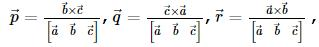then the value of the expression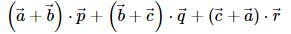equal to

Solution:
QUESTION: 25

The average of the number n sin n° for n = 2, 4, 6, ...180 is

Solution:
180/2 = 90.

The total no of terms = 90.

We can write the terms as:

(2 * sin(2) + 4 * sin(4) + 6 sin(6) + ... + 180 * sin(180)) / 90

as we know the relation sin θ = sin (180 - θ)

So, we can write: sin(2) = sin(178), sin(4) = sin(176) , all the way to sin(88) = sin(92)

And we know some of the values as well, like sin 180 is zero, sin90 is one and so on.

(2 * sin(2) + 178 * sin(2) + 4 * sin(4) + 176 * sin(4) + ... + 88 * sin(88) + 92 * sin(92) + 90 * sin(90)) / 90

or, (180 * (sin(2) + sin(4) + sin(6) + ... + sin(88)) + 90) / 90

Now most importantly, we have to know which deduction to be used where and we know sin/cos = tan. and cos/sin = cot.

So, the series can be written as sin(a) + sin(2a) + sin(3a) + ... + sin(na) = (1/2) * cot(a/2) - cos((n + 1/2) * a) / (2 * sin(a/2))

Therefore, sin(2) + sin(4) + ... + sin(88) =>

(1/2) * cot(2/2) - cos((44 + 1/2) * 2) / (2 * sin(2/2)) [ applying the above formula ]

or, (1/2) * (cos(1) - cos(89)) / sin(1)

(180 * (sin(2) + sin(4) + sin(6) + ... + sin(88)) + 90) / 90

or, (180 * (1/2) * (cos(1) - cos(89)) / sin(1) + 90) / 90

or, 90 * ((cos(1) - cos(89)) / sin(1) + 1) / 90

or, (cos(1) - cos(89) + sin(1)) / sin(1)

or, (cos(1) - sin(1) + sin(1)) / sin(1)

or, cos(1)/sin(1)

or, cot(1) = [RHS] Proved.

QUESTION: 26

The number of permutation of the letters PPPPQQQR in which the P's appear together in a block of four letters or the Q's appear in a block of 3 letters, is

Solution:
QUESTION: 27

Let f x be defined in R such that f (1) = 2, f (2) = 8 and f (u + v) = f (u) + kuv - 2v2 for all u , v ∈ R and k is a fixed constant. Then

Solution:

Explanation : f(1) = 2, f(2) = 8

f(u+v) = f(u) + kuv - 2v2

u = 1, v = 1

f(1+1) = f(1) +k(1)(1) - 2(1)2

f(2) = f(1) + k - 2

8 = 2 + k - 2

=> k = 8

f'(x) = lim(h-->0) [f(x+h) - f(x)]/h

= lim(h-->0) [f(x) + k*x*h - 2h2 - f(x)]/h

= lim(h-->0) h[kx - 2h]/h

f'(x) = kx - 2(0)

f'(x) = kx

f'(x) = 8x

QUESTION: 28

In the following question, a Statement of Assertion (A) is given followed by a corresponding Reason (R) just below it. Read the Statements carefully and mark the correct answer-

Assertion(A)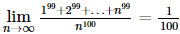Reason(R):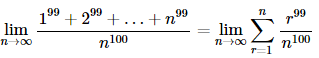Solution:
QUESTION: 29

In the following question, a Statement of Assertion (A) is given followed by a corresponding Reason (R) just below it. Read the Statements carefully and mark the correct answer-
Assertion(A): The orthocentre of a given triangle is coincident with the incentre of the pedal triangle of the given triangle.
Reason(R): Pedal triangle is the ex-central triangle of the given triangle.

Solution:
QUESTION: 30

In the following question, a Statement of Assertion (A) is given followed by a corresponding Reason (R) just below it. Read the Statements carefully and mark the correct answer-
Assertion(A):The determinants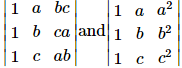are not identically equal.

Reason(R): If two rows (or columns) of a determinant are identical, then value of the determinant is zero.

Solution: